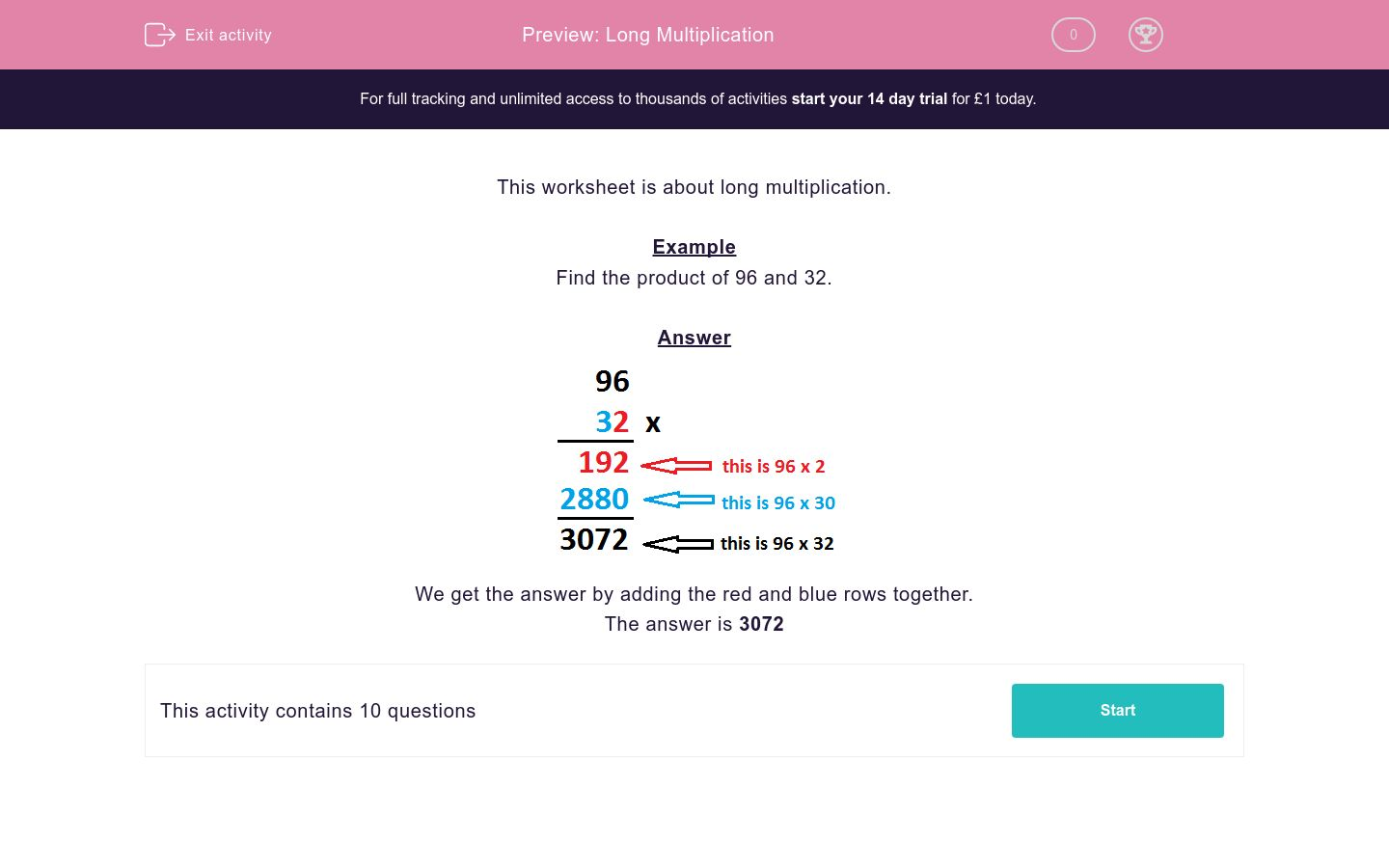# Long Multiplication

In this worksheet, students find the product of two two-digit numbers.Key stage:  KS 2

Curriculum topic:   Maths and Numerical Reasoning

Curriculum subtopic:   Mixed Problems

Difficulty level:### QUESTION 1 of 10

This worksheet is about long multiplication.

Example

Find the product of 96 and 32.We get the answer by adding the red and blue rows together.